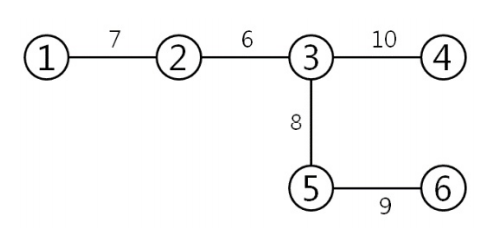시간 제한메모리 제한제출정답맞힌 사람정답 비율
2 초 256 MB127574443.564%

## 문제

The computer network in a country forms a tree, in other words, there is exactly one path between any two nodes. The weight of an edge (j,k), w(j,k), represents the time needed for sending a message from node j to node k. We assume w(j,k) = w(k,j). The length of the path between two nodes s and t is the sum of the weights of edges in the path between s and t. The diameter of a tree network is the length of the longest path among the paths between any two nodes in the network. For example, the diameter of the tree network in Figure 1 is 30 by the path between two nodes 1 and 6. The diameter is related to the longest delay in communicating between nodes in a network. So, diameter is one of important parameters in computer networks.Figure 1. A tree network

Now, assume we can reduce the communication time of an edge by paying a cost to the edge: By paying a cost to an arbitrary edge (j,k), we can reduce w(j,k) to max{w(j,k)-c, 0}. Here, the cost c can be any non-negative real number. We want to find the minimum cost such that the tree network has diameter at most D. For example, the minimum cost of the network above for a target diameter D = 26 is 4; by paying a cost 4 to the edge (5, 6), the weight 9 is reduced to 5. For a target diameter D = 0, the minimum cost is 40 because we should reduce all the weights of edges to 0. And, for a target diameter D = 19, the minimum cost is 11.5 by paying a cost 3.5 to the edge (2, 3), paying a cost 0.5 to the edge (3, 4), and paying a cost 7.5 to the edge (3, 5). You are to write a program, for a given tree network and a target diameter D, that computes the minimum cost.

## 입력

Your program is to read from standard input. The input consists of T test cases. The number of test cases T is given in the first line of the input. Each test case starts with a line containing two integer n (1 ≤ n ≤ 40,000) and D (0 ≤ D ≤ 50,000), where n is the number of nodes in the tree network and D is a target diameter. In the following n-1 lines, three integers representing an edge are given; the first and second integers represent end-nodes of the edge and the third integer represents the weight of the edge. Each edge weight is given as an integer between 1 and 50,000, inclusive. The node numbers are given as integers between 1 and n, inclusive. Note that although the initial edge weight is given as an integer, the weight can be reduced to a real number by paying a cost of real value.

## 출력

Your program is to write to standard output. For each test case, print the minimum cost so that the resulting tree network has diameter at most D. Your output should contain the first digit after the decimal point, rounded off from the second digit.

## 예제 입력 1

3
6 26
1 2 7
6 5 9
5 3 8
3 2 6
4 3 10
6 0
1 2 7
6 5 9
5 3 8
3 2 6
4 3 10
6 19
1 2 7
6 5 9
5 3 8
3 2 6
4 3 10


## 예제 출력 1

4.0
40.0
11.5